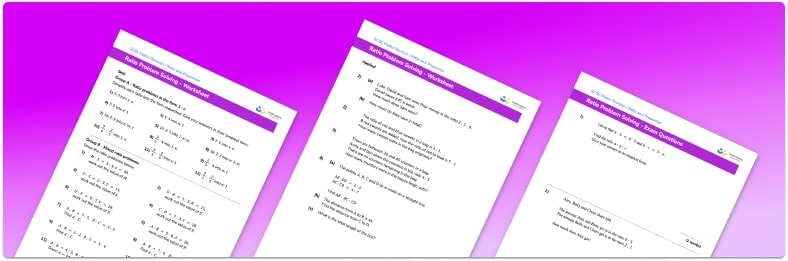# Ratio Problem Solving Worksheet• Section 1 of the ratio word problem worksheet contains 36 skills-based ratio word problem questions, in 3 groups to support differentiation
• Section 2 contains 4 applied ratio word problem questions with a mix of worded problems and deeper problem solving questions
• Section 3 contains 4 foundation and higher level GCSE exam style ratio word problem questions
• Answers and a mark scheme for all ratio word problem questions are provided
• Questions follow variation theory with plenty of opportunities for students to work independently at their own level
• All questions created by fully qualified expert secondary maths teachers
• Suitable for GCSE maths revision for AQA, OCR and Edexcel exam boards

• This field is for validation purposes and should be left unchanged.

You can unsubscribe at any time (each email we send will contain an easy way to unsubscribe). To find out more about how we use your data, see our privacy policy.

### Ratio problem solving at a glance

Ratios can be written as part to part or part to whole. This varied interpretation is necessary when solving worded problems.

Dividing a quantity by a given ratio:

The quantity is divided by the total sum of the parts of the ratio and then multiplied by each required part within the ratio.

Fraction word problems (and percents):

For the part to part ratio A:B, the fraction of the ratio that is A is equal to A (the numerator) divided by A+B (the denominator); the part to whole ratio A:C would be expressed as the fraction A divided by C. To convert the fraction to a percentage, we determine the equivalent fraction where the denominator is equal to 100.

Simplifying and equivalent ratios:

We can simplify ratios by dividing each part of the ratio by a common factor. Writing ratios in their simplest form makes it easier to visualise the relationship between the quantities in the ratios. It also makes it easier to use the ratios. Just as we can simplify ratios by dividing each part by a common factor, we can find other equivalent ratios by multiplying each part by a common number. We can use equivalent ratios to solve problems.

Ratio and proportion word problems:

Ratio word problems may ask us to write a ratio, simplify a ratio, divide a quantity into a ratio or use a ratio to find quantities. We can use a bar model to represent a given ratio and this can help us visualise the ratio problem more easily.

Rate word problems:

Writing a ratio in the form 1:n allows us to compare two quantities and determine their unit rate. This could be a currency conversion, the price per litre of fuel, or the speed of an object (compound measures).

Looking forward, students can then progress to additional ratio and proportion worksheets, for example a speed, distance, time worksheet or aFor more teaching and learning support on Ratio and Proportion our GCSE maths lessons provide step by step support for all GCSE maths concepts.

You will find free worksheets with real life problems and exam style questions for each topic. Each math worksheet is accompanied by an answer key.

When you have students who require more intensive support our one to one GCSE maths revision programme will match them with the most appropriate tutor. This way we can provide individual students with personalised programmes of study while you continue to teach the rest of your class as a whole group.

Our maths interventions are currently only available for GCSE students, and are not suitable for A level students.

## Do you have KS4 students who need more focused attention to succeed at GCSE?There will be students in your class who require individual attention to help them succeed in their maths GCSEs. In a class of 30, it’s not always easy to provide.

Help your students feel confident with exam-style questions and the strategies they’ll need to answer them correctly with our dedicated GCSE maths revision programme.

Lessons are selected to provide support where each student needs it most, and specially-trained GCSE maths tutors adapt the pitch and pace of each lesson. This ensures a personalised revision programme that raises grades and boosts confidence.

Find out more